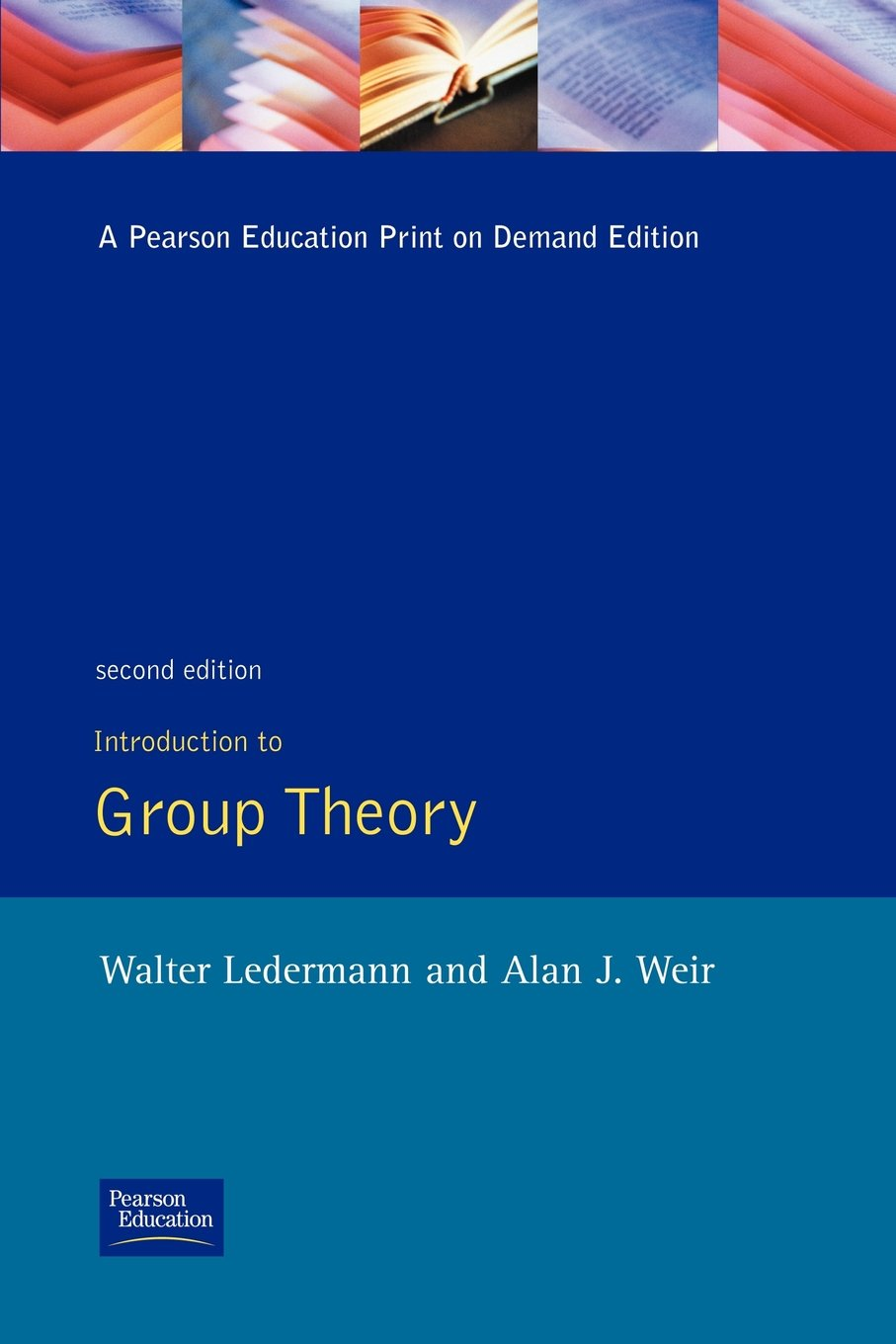The Cali Garmo

does Math

Introduction to Group Theory by Ledermann and Weir

By Cali G , Published on Mon 17 February 2014
Category: books / group theoryLedermann and Weir take a slightly unique approach in the theory of groups. Their text is slightly difficult to follow in a lot of places as they tend to group things together in non-standard ways. The biggest difference I found with his use of symbols is when talking about homomorphisms. In particular most texts will look at a homomorphism:$\theta : G \rightarrow G^{\prime}$ and when going from$G$ to$G^{\prime}$ they apply a function such as$\theta (g)$; Ledermann and Weird on the other hand choose to use the notation of$g\theta$. Although this format might be more concise, it can at times be a little confusing as to what each symbol is supposed to stand for. My assumption is that he's trying to show that a homomorphism is similar to group actions with the action being the map$\theta$, but for an introductory piece, it can be particularly confusing.